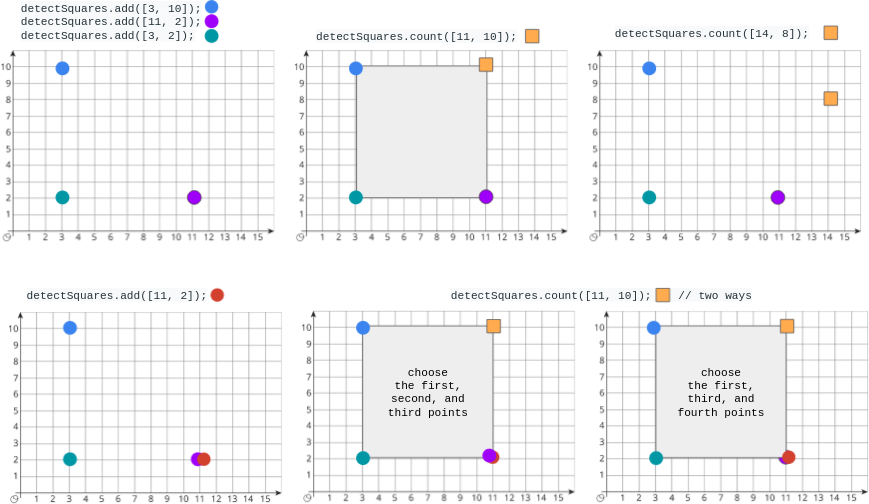# LeetCode 2013. Detect Squares Solution

You are given a stream of points on the X-Y plane. Design an algorithm that:

• Adds new points from the stream into a data structure.{" "} Duplicate points are allowed and should be treated as different points.
• Given a query point, counts the number of ways to choose three points from the data structure such that the three points and the query point form an axis-aligned square with{" "} positive area.

An axis-aligned square is a square whose edges are all the same length and are either parallel or perpendicular to the x-axis and y-axis.

Implement the DetectSquares class:

• DetectSquares() Initializes the object with an empty data structure.
• void add(int[] point) Adds a new point{" "} point = [x, y] to the data structure.
• int count(int[] point) Counts the number of ways to form{" "} axis-aligned squares with point{" "} point = [x, y] as described above.

Example 1:Input
"count"]{"\n"}[[], [[3, 10]], [[11, 2]], [[3, 2]], [[11, 10]], [[14, 8]],
[[11, 2]], [[11, 10]]]{"\n"}
Output
{"\n"}[null, null, null, null, 1, 0, null, 2]{"\n"}
{"\n"}
Explanation
{"\n"}DetectSquares detectSquares = new DetectSquares();{"\n"}
detectSquares.add([3, 2]);{"\n"}detectSquares.count([11, 10]); // return 1.
You can choose:{"\n"}
{"                               "}//{"   "}- The first, second, and third
points{"\n"}detectSquares.count([14, 8]);{"  "}// return 0. The query point
cannot form a square with any points in the data structure.{"\n"}
{"\n"}detectSquares.count([11, 10]); // return 2. You can choose:{"\n"}
{"                               "}//{"   "}- The first, second, and third
points{"\n"}
{"                               "}//{"   "}- The first, third, and fourth
points{"\n"}


Constraints:

• point.length == 2
• 0 <= x, y <= 1000
• At most 3000 calls in total will be made to{" "} add and count.

## Naive Solution

Store a list points of all existing points. To answer a query, loop through all triples of points and check if they form a square with the query point. This takes $\mathcal{O}(n^3)$ per query. $n$ can be up to $3000$, so this solutions is too slow.

## Full Solution

Notice that if we fix two opposite corners of a square, we've determined where the other two corners must be. Let our query point be p1. We loop through all points in points as candidates for p3, the opposite corner. We should ensure that the side lengths of the square are equal by checking that abs(p1.x-p3.x) = abs(p1.y-p3.y). We should also check that p1 and p3 are not the same point. Now we know that p2 = (p1.x, p3.y) and p4 = (p3.x, p1.y).

There could be multiple points at these locations, so we have a 2D array point_freq that stores how many points are at each (x, y) coordinate. In the add function, we increase the count of point_freq[point.x][point.y] by 1.

There are point_freq[p2] ways to choose a point at p2 and point_freq[p4] ways to choose a point at p4, so there are point_freq[p2] * point_freq[p4] ways to form a square with p1 and p3 as opposite corners. We add this to the answer of the current query.p1 is the query point. Each possible p3 uniquely determines the coorindates of p2 and p4.

### Time complexity

Each add operation takes $\mathcal{O}(1)$. Each query operation takes $\mathcal{O}(n)$ where $n$ is the number of existing points. $n \le 3000$, so our solution is more than fast enough.

### Space complexity

points takes $\mathcal{O}(n)$ space. point_freq is a 1001*1001 2D array, which can be considered a constant. If our coordinates were to exceed the range [0, 1000], we could use a hashmap instead, taking $\mathcal{O}(n)$ space.

## C++ Solution

1class DetectSquares {
2public:
3	int point_freq = {};
4	vector<pair<int, int>> points;
5
7		int x = point, y = point;
8		points.emplace_back(x, y);
9		point_freq[x][y]++;
10	}
11
12	int count(vector<int> point) {
13		int p1_x = point, p1_y = point;
14		int ans = 0;
15		for (auto [p3_x, p3_y]: points) {
16			if (p1_x != p3_x and abs(p1_x - p3_x) == abs(p1_y - p3_y)) {
17				ans += point_freq[p1_x][p3_y] * point_freq[p3_x][p1_y];
18				// p2 = (p1_x, p3_y) and p4 = (p3_x, p1_y)
19			}
20		}
21		return ans;
22	}
23};
24
25/**
26 * Your DetectSquares object will be instantiated and called as such:
27 * DetectSquares* obj = new DetectSquares();
29 * int param_2 = obj->count(point);
30 */

## Java Solution

1class DetectSquares {
2	int[][] point_freq = new int;
3	ArrayList<int[]> points = new ArrayList<>();
4
5	public void add(int[] p) {
6		int x = p, y = p;
8		point_freq[x][y]++;
9	}
10
11	public int count(int[] p) {
12		int p1_x = p, p1_y = p;
13		int ans = 0;
14		for (int[] p3 : points) {
15			int p3_x = p3, p3_y = p3;
16			if (p1_x != p3_x && Math.abs(p1_x - p3_x) == Math.abs(p1_y - p3_y)) {
17				ans += point_freq[p1_x][p3_y] * point_freq[p3_x][p1_y];
18				// p2 = (p1_x, p3_y) and p4 = (p3_x, p1_y)
19			}
20		}
21		return ans;
22	}
23}
24
25/**
26 * Your DetectSquares object will be instantiated and called as such:
27 * DetectSquares obj = new DetectSquares();
29 * int param_2 = obj.count(point);
30 */

## Python Solution

1class DetectSquares:
2	def __init__(self):
3		self.point_freq = [[0 for j in range(1001)] for i in range(1001)]
4		self.points = []
5
6	def add(self, point: List[int]) -> None:
7		x, y = point
8		self.point_freq[x][y] += 1
9		self.points.append(point)
10
11	def count(self, point: List[int]) -> int:
12		ans = 0
13		p1_x, p1_y = point
14		for [p3_x, p3_y] in self.points:
15			if p1_x != p3_x and abs(p1_x - p3_x) == abs(p1_y - p3_y):
16				ans += self.point_freq[p1_x][p3_y] * self.point_freq[p3_x][p1_y]
17				# p2 = (p1_x, p3_y) and p4 = (p3_x, p1_y)
18		return ans
19
20# Your DetectSquares object will be instantiated and called as such:
21# obj = DetectSquares()
23# param_2 = obj.count(point)